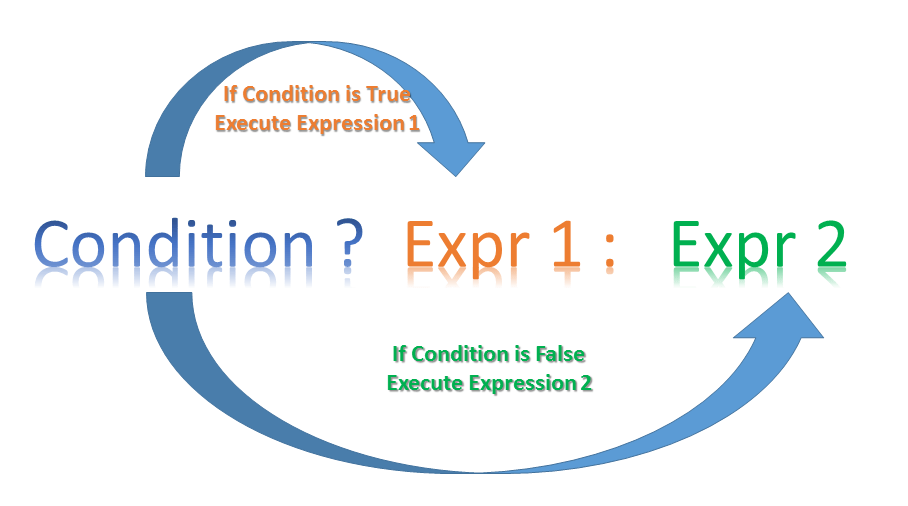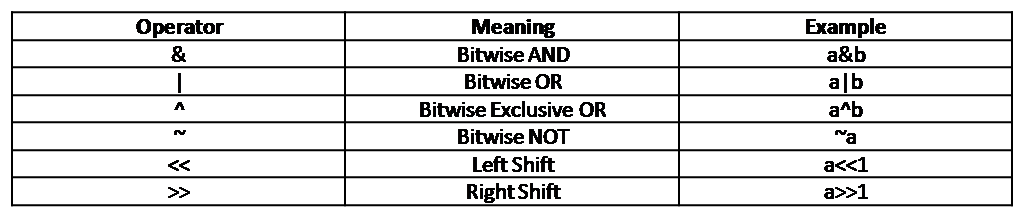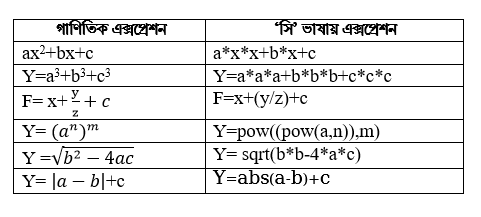Fifth Chapter Lesson-11 Operators and expressions of C language

At the end of this lesson-

• 1. You will be able to explain operators.
• 2. You will be able to describe different operators and their uses.
• 3. You will be able to explain expressions.
• 4. You will be able to explain the precedence and associativity of operators.

Operator: An operator is a symbol that tells the compiler to perform specific mathematical or logical operations.  In other words we can say that an operator operates the operands.The data items on which operators act upon are called operands.Operators are used in programs to manipulate constant and operands.

Consider the expression A + B * 5. where, +, * are operators, A, B  are operands, 5 is constant and A + B * 5 is an expression.

Depending on the number of operands that an operator can act upon, operators can be classified as follows:

• 1. Unary Operators
• 2. Binary Operators
• 3. Ternary Operators

Unary Operators:Those operators that require only single operand to act upon are known as unary operators.For Example increment(++) and decrement(–) operators.

Binary Operators:Those operators that require two operands to act upon are called binary operators.Binary operators are classified into :

• 1. Arithmetic operators (+, -, * etc.)
• 2. Relational Operators ( <, >, ==)
• 3. Logical Operators (&&, ||)
• 4. Assignment Operators (=, +=, -=)
• 5. Bitwise Operators (&, |)

Ternary Operators: These operators requires three operands to act upon. For Example Conditional operator(?:).

C operators can be classified into following types based on operations:

• 1. Arithmetic operators
• 2. Relational operators
• 3. Logical operators
• 4. Assignment operators
• 5. Increment/decrement operators
• 6. Conditional operators
• 7. Bitwise operators
• 8. Special operators

Arithmetic operators:These operators are used to perform mathematical calculations on operands like addition, subtraction, multiplication, division and modulus.

 Operators Description + Adds two operands. − Subtracts second operand from the first. * Multiplies both operands. / Divides numerator by de-numerator. % Modulus Operator and remainder of after an integer division.

Relational operators: These operators are used to compare the value of two operands. For example: checking if one operand is equal to the other operand or not, an operand is greater than the other operand or not etc.

 Operators Description Example == Checks if the values of two operands are equal or not. If yes, then the condition becomes true. (A == B) is not true. != Checks if the values of two operands are equal or not. If the values are not equal, then the condition becomes true. (A != B) is true. > Checks if the value of left operand is greater than the value of right operand. If yes, then the condition becomes true. (A > B) is not true. < Checks if the value of left operand is less than the value of right operand. If yes, then the condition becomes true. (A < B) is true. >= Checks if the value of left operand is greater than or equal to the value of right operand. If yes, then the condition becomes true. (A >= B) is not true. <= Checks if the value of left operand is less than or equal to the value of right operand. If yes, then the condition becomes true. (A <= B) is true.

Logical operators: Logical Operators are used to combine two or more conditions/constraints or to complement the evaluation of the original condition in consideration. The result of the operation of a logical operator is a boolean value either true or false.

 Operator Description Example && Called Logical AND operator. If both the operands are non-zero, then the condition becomes true. (A && B) is false. || Called Logical OR Operator. If any of the two operands is non-zero, then the condition becomes true. (A || B) is true. ! Called Logical NOT Operator. It is used to reverse the logical state of its operand. If a condition is true, then Logical NOT operator will make it false. !(A && B) is true.

Assignment operators: These operators are used to assign the values for the variables in C programs. The left side operand of the assignment operator is a variable and right side operand of the assignment operator is a value. The value on the right side must be of the same data-type of variable on the left side otherwise the compiler will raise an error.

 Operator Description Example = Simple assignment operator. Assigns values from right side operands to left side operand C = A + B will assign the value of A + B to C += Add AND assignment operator. It adds the right operand to the left operand and assign the result to the left operand. C += A is equivalent to C = C + A -= Subtract AND assignment operator. It subtracts the right operand from the left operand and assigns the result to the left operand. C -= A is equivalent to C = C – A *= Multiply AND assignment operator. It multiplies the right operand with the left operand and assigns the result to the left operand. C *= A is equivalent to C = C * A /= Divide AND assignment operator. It divides the left operand with the right operand and assigns the result to the left operand. C /= A is equivalent to C = C / A %= Modulus AND assignment operator. It takes modulus using two operands and assigns the result to the left operand. C %= A is equivalent to C = C % A

Increment/decrement operators: Increment Operators are used to increased the value of the variable by one and Decrement Operators are used to decrease the value of the variable by one in C programs. Both increment and decrement operator are used on a single operand or variable, so it is called as a unary operator. Unary operators are having higher priority than the other operators it means unary operators are executed before other operators.

Type of Increment Operator

• pre-increment
• post-increment

pre-increment (++ variable): In pre-increment first increment the value of variable and then used inside the expression (initialize into another variable).

Output:

post-increment (variable ++): In Post-increment first value of variable is used in the expression (initialize into another variable) and then increment the value of variable.

Output:

Type of Decrement Operator

• pre-decrement
• post-decrement

Pre-decrement (– variable): In pre-decrement first decrement the value of variable and then used inside the expression (initialize into another variable).

Output:

post-decrement (variable –): In Post-decrement first value of variable is used in the expression (initialize into another variable) and then decrement the value of variable.

Output:

Conditional operators: Conditional operators return one value if condition is true and returns another value is condition is false. This operator is also called as ternary operator.

Syntax     :        (Condition? true_value: false_value);
Example :         (A < 0  ? Negative  :  Positive);
In above example, if A is less than 0, Negative is returned else Positive is returned. This is equal to if else conditional statements.Bitwise operators: These operators are used to perform bit-level operations on the operands. The operators are first converted to bit-level and then calculation is performed on the operands. Bitwise operators can not be used on float and double type data.Truth table of bitwise operators:Special operators: Below are some of the special operators that the C programming language offers.

 Operators Description & This is used to get the address of the variable.Example : &a will give address of a. * This is used as pointer to a variable.Example : * a  where, * is pointer to the variable a. Sizeof () This gives the size of the variable.Example : size of (char) will give us 1.

Expression: Operators, functions, constants and variables are combined together to form expressions. Consider the expression A + B * 5. where, +, * are operators, A, B  are variables, 5 is constant and A + B * 5 is an expression.

Mathematical expressions are written in c program in the following way:Operator precedence and associativity in expression:

Operator precedence determines which operator is performed first in an expression with more than one operators with different precedence. For example 10 + 20 * 30 is calculated as 10 + (20 * 30) and not as (10 + 20) * 30.

Associativity is used when two operators of same precedence appear in an expression. Associativity can be either Left to Right or Right to Left. For example ‘*’ and ‘/’ have same precedence and their associativity is Left to Right, so the expression “100 / 10 * 10” is treated as “(100 / 10) * 10”.

Here, operators with the highest precedence appear at the top of the table, those with the lowest appear at the bottom. Within an expression, higher precedence operators will be evaluated first.

CategoryOperatorAssociativity
Postfix() [] -> . ++ – –Left to right
Unary+ – ! ~ ++ – – (type)* & sizeofRight to left
Multiplicative* / %Left to right
Additive+ –Left to right
Shift<< >>Left to right
Relational< <= > >=Left to right
Equality== !=Left to right
Bitwise AND&Left to right
Bitwise XOR^Left to right
Bitwise OR|Left to right
Logical AND&&Left to right
Logical OR||Left to right
Conditional?:Right to left
Assignment= += -= *= /= %=>>= <<= &= ^= |=Right to left
Comma,Left to right

Lesson Evaluation-

Knowledge Based Questions:

• a. What is operator?
• a. What is unary operator?
• a. What is binary operator?
• a. What is ternary operator?
• a. What is relational operator?
• a. What is logical operator?
• a. What is conditional operator?
• a. What is expression?
• a. What is operand?
• a. What is operator precedence?

Comprehension Based Questions:

• b. When unary operator is used? explain.
• b. Write the importance of operator in program.
• b. Explain i++ and ++i.
• b. Write differences between ‘=’ and ‘==’ .

Creative Questions:

Multiple Choice Questions:

Written by,